1800-1023-196

+91-120-4616500

# Heat of Reaction in an Electrochemical Cell

## Heat of Reaction in an Electrochemical Cell

Let n Faraday charge flows out of a cell of emf E, then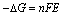…….(i)

Gibbs – Helmholtz equation from thermodynamics may be given as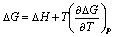…….(ii)

From equation (i) and (ii) we get,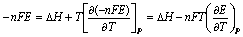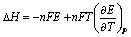where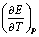= Temperature coefficient of cell

Case I: When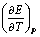= 0, then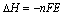Case II: When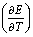> 0, then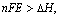i.e. process inside the cell is endothermic.

Case III: When< 0, then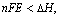i.e., process inside the cell is exothermic.

## NEET & AIIMS Exam Sample Papers

 AIIMS SAMPLE PAPERS View More NEET SAMPLE PAPERS View More